Next: Analytical calculation: ANB Up: Treatment of experimental deficiencies Previous: Treatment of experimental deficiencies

## Monte Carlo simulation: MCB

Measured electron beam parameters and their standard deviation as well as radiator and collimator properties are the basic input for calculations based on the Monte Carlo technique. Starting from a given number of electrons Ne, depending on the desired statistical accuracy, a certain set of physical values are chosen randomly in parameter space. First the direction of an incident electron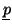with energy E0 impinging at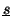on the radiator is chosen from the beam energyand divergence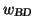distributions, which are assumed to be of Gaussian shape with known parameters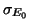,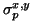and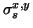respectively. The mean polar angle deviation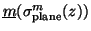from the incident direction depend via Molières theory on the depth z of the bremsstrahl process in the radiator, which is chosen randomly from a homogenous distribution within the radiator thickness zR. To calculate the coherent bremsstrahlung for this particular electron the lattice has to be rotated into its coordinate system, involving a transformation of the crystal angles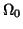. The total transversal electron deflection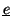due to multiple scattering and beam divergence and the transformation of the crystal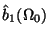axis in the electron system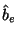is calculated (eq. A5c and fig. 2). Then a lattice vector is chosen uniformly in reciprocal space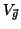with the Miller indices h,k,l, the intensity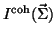is calculated with these parameters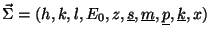and the photon momentum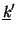is transformed back in the lab system. The resulting cross section is differential in photon energy k and angle, which is the azimuthal (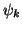) in coherent bremsstrahlung and is the polar angle (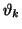) in the incoherent case. As an example the polarisation for a rectangular collimator compared to a circular one, both producing the same tagging efficiency, is shown in fig. 3.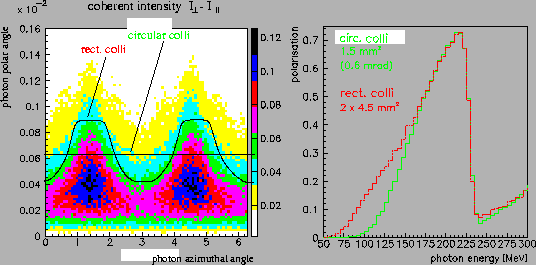Next: Analytical calculation: ANB Up: Treatment of experimental deficiencies Previous: Treatment of experimental deficiencies
Frank Natter
1999-07-16